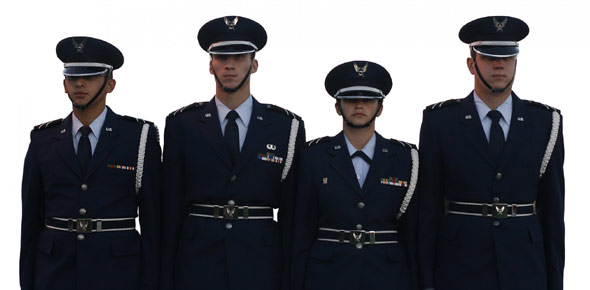# 2A6X1 Vol. 2 Ure's (Mfe)

100 Questions | Total Attempts: 271SettingsEngines eoc study guide

• 1.
"An unbalanced foce ona body produces or tends to produce an acceleration in the direction of the force and that the acceleration, if any, is directly proportional to the force and inversely proportional to the mass of the body" This statement is
• A.

Bernoulli's principle

• B.

Newton's first law of motion

• C.

Newton's second law of motion

• D.

Newton's third law of motion

• 2.
Which type of duct would decrease the velocity and increase the pressure of a gas as it passes through?
• A.

Elbow

• B.

Straight

• C.

Divergent

• D.

Convergent

• 3.
"The combination of a decreased pressure above an airfoil, and the increased pressure below the airfoil produces lift" ids best described by
• A.

Bernoulli's principle

• B.

Newton's first law of motion

• C.

Newton's second law of motion

• D.

Newton's third law of motion

• 4.
The tendency of an object at rest to remain at rest and the tendency of an object in motion to continue in motion in a straight line at the same speed is called
• A.

Energy

• B.

Inertia

• C.

Friction

• D.

Potential

• 5.
Which section of a jet engine introduces and burns fuel?
• A.

Turbine

• B.

Diffuser

• C.

Compressor

• D.

Combustion

• 6.
Which section of a jet engine keeps the compressor rotating?
• A.

Turbine

• B.

Exhaust

• C.

Diffuser

• D.

Combustion

• 7.
Which method of producing thrust does a turboprop engine use?
• A.

Accelerating a large mass of air by a small amount

• B.

Moving a small quantity of air through a large velocity change.

• C.

Using the same working fluid for propulsive force as that used within the engine

• D.

Using only the energy expended through the exhaust nozzle to propel the aircraft forward

• 8.
Which type of horsepower is delivered to the propeller for useful work?
• A.

Brake

• B.

Indicated

• C.

Frictional

• D.

Equivalent shaft

• 9.
The heat content per pound of kerosene is how many BTU's?
• A.

10,500

• B.

15,500

• C.

18,500

• D.

40,000

• 10.
To measure barometric pressure, you would use a
• A.

Potentiometer

• B.

Psychrometer

• C.

Pyrometer

• D.

Barometer

• 11.
What is the sea-level atmosperic pressure at "standard day" conditions?
• A.

13.5 psi

• B.

14.7 psi

• C.

49.94 psi

• D.

40.55 psi

• 12.
What effect does the divergent design of a diffuser have on air pressure?
• A.

Increases pressure

• B.

Decreases pressure

• C.

Has no effect on pressure

• D.

Results in pressure fluctuations

• 13.
Which engine component meters fuel for combustion?
• A.

P&D valve

• B.

Fuel pump

• C.

Fuel control

• D.

Fuel nozzles

• 14.
What directs the gases onto the first-stage turbine wheel blades in a jet engine?
• A.

Flameholder

• B.

Combustion chamber

• C.

• D.

Turbine stator

• 15.
When two or more turbine wheels are used in a jet engine, which component is placed directly in front of each turbine wheel?
• A.

Diffuser

• B.

Jet nozzle

• C.

Combustion chamber

• D.

Turbine stator(nozzle diaphragm

• 16.
Three major sections of all jet engines are compressor, combustion, and
• A.

Turbo

• B.

Turbine

• C.

Exhaust

• D.

Diffuser

• 17.
Vane-type fuel pumps used in jet engines are similar to
• A.

Sliding-vane air compressors

• B.

Piston pump air compressors

• C.

Roots air compressors

• D.

Turbo-superchargers

• 18.
The two forces that air is subjected to after it is drawn into the gude vanes of a centrifugal compressor are
• A.

Rotational and centrifugal

• B.

Rotational and tangential

• C.

Tangential and centrifugal

• D.

• 19.
A constructional feature of the centrifugal compressor is that the impeller
• A.

Has two matching plates

• B.

Has a lightweight design

• C.

Is a forged single unit

• D.

Is constructed in rows of blades and stators

• 20.
The forward end of the shaft on a centrifugal compressor is hollow in order to
• A.

Act as a vibation damper

• B.

Allow cooling air to the turbine

• C.

Provide a passage for lubricating oil

• D.

Provide a passage for the main fuel supply

• 21.
Stationary vanes positioned between rotor discs in a compressor are used to
• A.

Direct air and increase pressure

• B.

Direct hot gases rearward

• C.

Increase backup pressure

• D.

Prolong compressor life

• 22.
In a dual-spool compressor, rpm o the N2 compressor are determined by the
• A.

Starter

• B.

Fuel pump

• C.

Fuel control

• D.

Low-pressure turbine

• 23.
Which facet of engine design helps prevent igniter plug fouling?
• A.

Gold plating on the plug

• B.

Split electrode on the plug

• C.

Ceramic coating on the plug

• D.

Directing air around the plug

• 24.
The excess airflow that is not burned in the combustion section is used to
• A.

Cool the burner surfaces

• B.

Operate pneumatic accessories

• C.

Decrease the mass of exhaust gases

• D.

Increase air in compressor section

• 25.
Fuel that accumulates after a failed start is
• A.

Returned to the fuel control by tubes

• B.

Drained overboard by a drain system

• C.

Burned on the next start attempt

• D.

Allowed to evaporate

Related TopicsBack to top